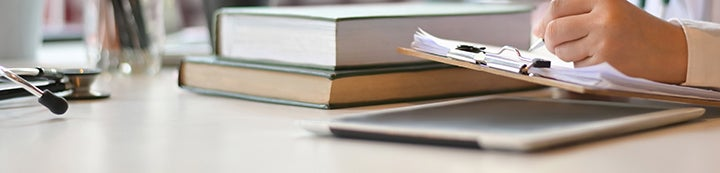1 - 5 of 5 Posts

#### TAAOM

·
##### Registered
Joined
·
103 Posts
Discussion Starter · ·
hey ppl plzzz help me out with this ......how to determine the power of a study ? ....how to calculate it?....i cant figure it out :O pllzzzzz helpppp!!!

#### rasheed

·
##### Registered
Joined
·
741 Posts
1 - type II error

The power of a statistical test is the probability that the test will reject a false null hypothesis (i.e. that it will not make a Type II error). As power increases, the chances of a Type II error decrease. The probability of a Type II error is referred to as the false negative rate (β). Therefore power is equal to 1 − β, which is equal to sensitivity.

•Carolina_USMLE

#### rasheed

·
##### Registered
Joined
·
741 Posts
Don't confuse it with accuracy

The accuracy of the study is different.

It's calculated by the following formula:

Accuracy = TP + TN / TP + FP + TN + FN

#### TAAOM

·
##### Registered
Joined
·
103 Posts
Discussion Starter · ·
thxxxx sooo much for ur reply rasheed .....n plzzz can u explain it with an example

#### bebix

·
##### Registered
Joined
·
1,357 Posts
The power of a statistical test is the probability that the test will reject a false null hypothesis (i.e. that it will not make a Type II error). As power increases, the chances of a Type II error decrease. The probability of a Type II error is referred to as the false negative rate (β). Therefore power is equal to 1 − β, which is equal to sensitivity.
Therefore power is equal to 1 − β, which is equal to sensitivity??

1 - 5 of 5 Posts